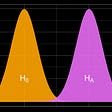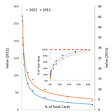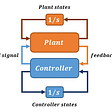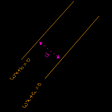# The basics of complex number arithmetic

The so-called “complex numbers” are actually quite simple. The terminology surrounding the complex numbers reflects centuries of ignorance as humans groped for an understanding of how to quantify things that are not solid.

In the study of electricity and in the study of particle physics, it is often necessary to deal with a “special” number x that is a solution to the equation x² = −1, or multiples of that number.

The similar equation x² = 1 is quite easy to solve. It has two solutions and you already know the most obvious solution. The other solution, though less obvious, takes only a little thought to figure out: x = −1 (every high school graduate should be able to figure it out).

That’s because a negative number times a negative number is a positive number. So −1 times −1 equals 1. Indeed any negative number times any other negative number is positive, and any positive number times any other positive number is also positive.

Clearly x² = 0 if x = 0, so x² is a positive number if x is any number from that familiar number line that includes the numbers −1, 0 and 1. It looks like x² = −1 has no solutions.

It’s lost to history who first thought to simply imagine that a solution exists. We can call this solution the “imaginary” unit and notate it i, or j as electrical engineers prefer (I’ll use i for the rest of this article — we won’t be delving into the mathematics of electricity in this article).

Then we can deal with this sort of unknown quantity as we deal with other unknown quantities in algebra. Twice the imaginary unit is notated 2i. And the imaginary unit negated is (−1) × i = −i, or, as mathematicians prefer to write it, (−1)i = −i.

Similarly, we see that (−i)² = (−1)²i² = −1. So the equation x² = −1 in fact has two solutions, just like the x² = 1 equation. Indeed any equation of the form x² + c, where c is some specific number, has two solutions.

The practical applications of imaginary numbers are quite real, but the terminology has stuck. The disadvantages of changing the terminology far outweigh the advantages.

So far we’ve only dealt with multiplication. Arithmetic of course also requires addition, and that’s as easy as additions with a single unknown quantity.

Maybe you vaguely remember from high school algebra that you solved simple “problems” like (5 + 2x) + (3 − 12x). All you have to do is line up the multiples of x and go from there. The answer in the example is then simply (5 + 3) + (2x − 12x) = 8 − 10x.

Suppose that x = i. Then we have (5 + 2i) + (3 − 12i) = (5 + 3) + (2i − 12i) = 8 − 10i. Easy enough. You can figure out subtraction from this (I’ll still post the formula at the end).

Sometimes a number like 1 is called a “purely real” number and a number like i is called a “purely imaginary” number, distinguishing them from “proper” or “nontrivial” complex numbers that have nonzero real part and nonzero imaginary part, like 8 − 10x.

It can nevertheless be useful to regard all numbers as complex and fill in 0 or 0i as needed, e.g., (8 − 10i) + 1 = (8 − 10i) + (1 + 0i) = 9 − 10i and (8 − 10i) + i = (8 − 10i) + (0 + i) = 8 − 9i.

Though I admit that’s much more useful for division than for addition or multiplication. Before we can deal with the division of complex numbers, we need to understand the multiplication of “proper” complex numbers.

To keep this next example simple, I’m going to make the multiplicands only have positive real parts and positive imaginary parts. Here we go: (2 + i)(3 + 5i). The result will probably have negative real or negative imaginary parts.

Multiplication is distributive, meaning that (a + b)c = ac + bc. But that doesn’t seem to help us here, because we need (a + b)(c + d). I still remember the mnemonic FOIL: First, Outer, Inner, Last.

Then, given (2 + x)(3 + 5x), we have 6 + 10x + 3x + 5x², which we can simplify to 6 + 13x + 5x². But we want x = i, and since i² = −1, we can simplify 6 + 13i + 5i² further: 6 + 13i + 5i² = 6 + 13i − 5 = 1 + 13i.

From FOIL we can glean the formula (a + bx)(c + dx) = ac + adx + bcx + bdx² = ac + (ad + bc)x + bdx². But if we have x = i, then ac + (ad + bc)i + bdi² can be simplified to (acbd) + (ad + bc)i.

Of course if any of a, b, c or d are negative, you will have to take care to use the right signs or your answer might not be correct. That’s why I gave the example with only positive real parts and positive imaginary parts.

I recommend you try out on your own an example with negative real parts and/or negative imaginary parts.

Things get extremely complicated for division, so I’m just going to give you the formula without explaining how to get the formula.

But I’m not asking you to take the formula on faith: if you know how to multiply two complex numbers, you also know how to verify the division of a complex number by another complex number.

The formula to divide a + bi by c + di is (ac + bd + (bcad)i)/(c² + d²). If that’s correct, using it to calculate 1 + 13i divided by 2 + i should give 3 + 5i, and likewise 1 + 13i divided by 3 + 5i should give 2 + i, since those are the numbers we multiplied to get 1 + 13i.

Plugging in the former into the formula, we get (2 + 13 + (26 − 1)i)/(4 + 1) = (15 + 25i)/5 = 3 + 5i. That checks out. You can check the latter, 1 + 13i divided by 3 + 5i, if you want.

# A note about division by zero

Even with complex numbers, division by zero is still invalid, undefined, whatever you want to call it. Just don’t call it well-defined.

We’re going to set the “properly” imaginary and “properly” complex numbers aside for a moment and focus on purely real numbers (technically zero is both purely real and purely imaginary).

Given a purely real positive divisor d such that d > 1, we see that 1/d < 1. For example, 1/5 = 0.2. Obviously if d = 1, then 1/d = 1. And if d < 1, but with d still positive, we see that 1/d > 1. For example, 1/0.2 = 5.

As we make d smaller still, and closer to 0, but still greater than 0, 1/d will be even larger and closer to positive infinity. For example, 1/0.0000000001 = 10000000000. Surely that must mean that 1/0 equals positive infinity.

If you think that makes sense, then you need to look at it from the other side. Given a purely real negative divisor d such that d < −1, we see that 1/d > −1.

For example, 1/−5 = −0.2. Obviously if d = −1, then 1/d = −1. And if d > −1, but with d still negative, we see that 1/d < −1. For example, 1/−0.2 = −5. Hopefully I haven’t made any mistakes with the comparison operators in these examples.

As we make d smaller still in absolute value, and closer to 0, but still less than 0, 1/d will be even larger in absolute value and closer to negative infinity. Surely that must mean that 1/0 equals negative infinity.

That contradicts my earlier assertion that 1/0 equals positive infinity. I’m guessing that’s what mathematicians call a “discontinuity.”

A similar situation occurs with i/d where d is purely real. As d gets closer to 0, i/d approaches positive infinity times i if d > 0 and negative infinity times i if d < 0. And if d is allowed to have a nonzero imaginary part, you can send division by zero in whatever direction you want. Just choose the angle you want relative to the real number line.

However, it’s perfectly valid to divide any complex number by a number with real part zero or imaginary part zero if the other part is not zero.

Notice the c² + d² in the division formula: if either c or d is a nonzero number, or if they are both nonzero, then c² + d² is positive, and there is no problem of division by zero.

# Summary

Let’s review the formulas for the basic arithmetic of complex numbers:

• (a + bi) + (c + di) = (a + c) + (b + d)i. For example, (1 + i) + (7 + 13i) = 8 + 14i.
• (a + bi) − (c + di) = (ac) + (bd)i. For example, (1 + i) − (7 + 13i) = −6 − 12i.
• (a + bi)(c + di) = (acbd) + (ad + bc)i. For example, (2 − 7i)(2 + 7i) = 53 + 0i.
• (a + bi)/(c + di) = ((ac + bd) + (adbc)i)/(c² + d²). For example, (53 + 0i)/(2 + 7i) = 2 − 7i.

Well, there you have it, complex number arithmetic is actually quite simple. At least as far as the basic arithmetic operations are concerned. And division by zero is just as invalid and undefined as when we only deal with purely real numbers.

Even so, you might prefer to use a computer to do calculations with complex numbers. As long as the computer has been properly programmed, and with caveats for numeric precision, the computer should give correct results.

--

--

--

## More from Alonso Del Arte

is a composer and photographer from Detroit, Michigan. He has been working on a Java program to display certain mathematical diagrams.

Love podcasts or audiobooks? Learn on the go with our new app.

## A/B Test Statistics Made Easy## The Equals Sign is a Myth## Pareto Principle and Gini Coefficient explained in Terms of Trading Cards## What Happens When Maths Goes Wrong? — with Matt Parker## Why We Need to Change the Way Math Is Taught?## MATHEMATICS IN THE 21ST CENTURY## Alonso Del Arte

is a composer and photographer from Detroit, Michigan. He has been working on a Java program to display certain mathematical diagrams.

## Histograms in Layman’s terms## Control Theory: Separation principle and the Symbiosis between Controller and Plant## Distances between two hyper planes